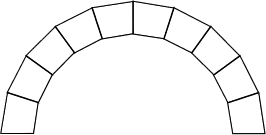#### You may also like### Getting an Angle

How can you make an angle of 60 degrees by folding a sheet of paper twice?### Arclets Explained

This article gives an wonderful insight into students working on the Arclets problem that first appeared in the Sept 2002 edition of the NRICH website.### Bow Tie

Show how this pentagonal tile can be used to tile the plane and describe the transformations which map this pentagon to its images in the tiling.

# Trapezium Arch

##### Age 11 to 14 ShortChallenge LevelTen stones, of identical shape and size, are used to make an arch, as shown in the diagram. Each stone has a cross-section in the shape of a trapezium with equal sides. What is the size of the smallest angles of the trapezium?

If you liked this problem, here is an NRICH task which challenges you to use similar mathematical ideas.

This problem is taken from the UKMT Mathematical Challenges.
You can find more short problems, arranged by curriculum topic, in our short problems collection.